### 23.2 Passing C++ memory to Python

To highlight the technique for passing C++ memory natively into Python’s `ndarray`, we consider first the case of the native Nektar++ matrix structure. In many situations, matrices created by Nektar++ (usually a `shared_ptr` of `NekMatrix<D, StandardMatrixTag>` type) need to be passed to Python - for instance, when performing differentiation using e.g. Gauss quadrature rules a differentiation matrix must be obtained. In order to keep the program memory efficient, the data should not be copied into a NumPy array but rather be referenced by the Python interface. This, however, complicates the issue of memory management.

Consider a situation where C++ program no longer needs to work with the generated array and the memory dedicated to it is deallocated. If this memory has already been shares with Python, the Python interface may still require the data contained within the array. However since the memory has already been deallocated from the C++ side, this will typically cause an out-of-bounds memory exception. To prevent such situations a solution employing reference counting must be used.

##### Converter method

Boost.Python provides the methods to convert a C++ type element to one recognised by Python as well as to maintain appropriate reference counting. Listing 23.1 shows an abridged version of the converter method (for Python 2 only) with comments on individual parameters. The object requiring conversion is a `shared_ptr` of `NekMatrix<D, StandardMatrixTag>` type (named `mat`).

Listing 23.1: Converter method for converting the C++ arrays into Python NumPy arrays.
1#include <LibUtilities/LinearAlgebra/NekMatrix.hpp>
2#include <LibUtilities/LinearAlgebra/MatrixStorageType.h>
3#include <NekPyConfig.hpp>
4
5using namespace Nektar;
6using namespace Nektar::LibUtilities;
7
8template<typename T, typename F>
9void NekMatrixCapsuleDestructor(void *ptr)
10
11    // Destructor for shared_ptr when the capsule is deallocated.
12    std::shared_ptr<NekMatrix<T, F>> *mat =
13        (std::shared_ptr<NekMatrix<T, F>> *)ptr;
14    delete mat;
15
16
17template<typename T>
18struct NekMatrixToPython
19
20    static PyObject *convert(
21        std::shared_ptr<NekMatrix<T, StandardMatrixTag>> const &mat)
22    {
23        // Create a PyCObject, which will hold a shared_ptr to the matrix.
24        // When capsule is deallocated on the Python side, it will call the
25        // destructor function above and release the shared_ptr reference.
26        py::object capsule(py::handle<>(PyCObject_FromVoidPtr(
27            new std::shared_ptr<NekMatrix<T, StandardMatrixTag>>(mat),
28            NekMatrixCapsuleDestructor<T, StandardMatrixTag>)));
29
30        int nRows = mat->GetRows(), nCols = mat->GetColumns();
31
32        // increments Python reference counter to avoid immediate deallocation
33        return py::incref(
34            np::from_data(
35                mat->GetRawPtr(), // pointer to data
36                np::dtype::get_builtin<T>(), // data type
37                py::make_tuple(nRows, nCols), // shape of the array
38                py::make_tuple(sizeof(T), nRows * sizeof(T)), // stride of the array
39                capsule).ptr()); // capsule - contains the object owning the data (preventing it from being prematurely deallocated)
40    }
41
42
43template<typename T>
44void export_NekMatrix()
45
46    py::to_python_converter<std::shared_ptr<NekMatrix<T, StandardMatrixTag>>,
47                            NekMatrixToPython<T>>();
48}

Firstly we give a brief overview of the general process undertaken during type conversion. Boost.Python maintains a registry of known C++ to Python conversion types, which by default allows for fundamental data type conversions such as `double` and `float`. In this manner, many C++ functions can be automatically converted, for example when they are used in `.def` calls when registering a Python class. Clearly the `convert` function here contains much of the functionality. In order to perform automatic conversion between the `NekMatrix` and a 2D `ndarray`, we register the conversion function inside Boost.Python’s registry so that it is aware of the datatypes. We also note that throughout the conversion code (and elsewhere in NekPy), we make use of the Boost.NumPy bindings. These are a lightweight wrapper around the NumPy C API, which simplifies the syntax somewhat and avoids direct use of the API.

In terms of the conversion function itself, we first create a new Python capsule object. The capsule is designed to hold a C pointer and a callback function that is called when the Python object is deallocated. Since there is no Boost.Python wrapper around this, we use a `handle<>` to wrap it in a generic Boost.Python object. The strategy we therefore employ is to create a `shared_ptr`, which increases the reference counter of the `NekMatrix`. This will prevent it being destroyed if it is in use in Python, even if on the C++ side the memory is deallocated. The callback function simply deletes the `shared_ptr` when it is no longer required, cleaning up the memory appropriately. This process is shown in Figure 23.3. It is worth noting that the steps (c) and (d) can be reversed and the `shared_ptr` created by the Python binding can be removed first. In this case the memory will be deallocated only when the `shared_ptr` created by C++ is also removed.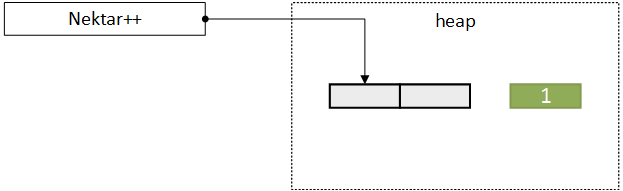(a) Nektar++ creates a data array referenced by a `shared_ptr`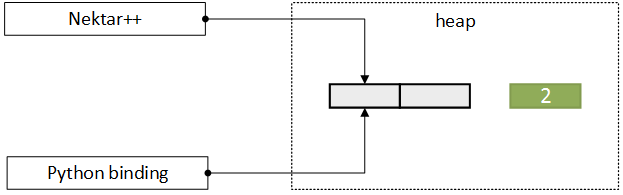(b) Array is passed to Python binding which creates a new `shared_ptr` to the data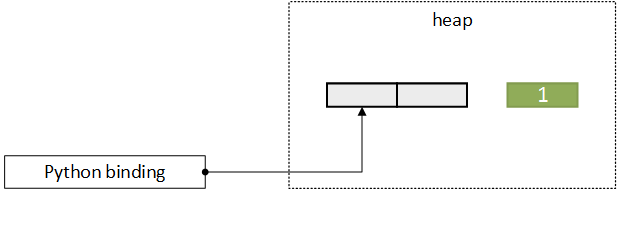(c) Nektar++ no longer needs the data - its `shared_ptr` is removed but the memory is not deallocated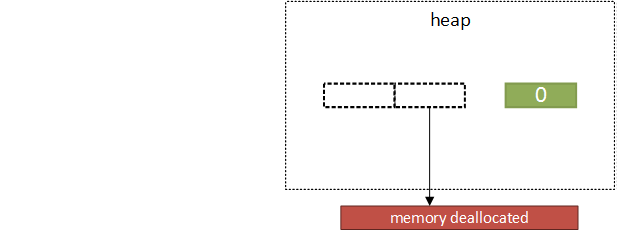(d) When the data is no longer needed in the Python interface the destructor is called and `shared_ptr` is removed.

Figure 23.3 Memory management of data created in C++ using `shared_ptr` and passed to Python. Reference counter shown in green.

Finally, the converter method returns a NumPy array using the `np::from_data` method. Note that the `capsule` object is passed as the `ndarray` base argument – i.e. the object that owns the data. In this case, when all `ndarray` objects that refer to the data become deallocated, NumPy will not directly deallocate the data but instead release its reference to the capsule. The capsule will then be deallocated, which will decrement the counter in the `shared_ptr`. We also note that data ordering is important for matrix storage; Nektar++ stores data in column-major order, whereas NumPy arrays are traditionally row-major. The stride of the array has to be passed into the `np::from_data` in a form of a tuple `(a, b)`, where `a` is the number of bytes needed to skip to get to the same position in the next row and `b` is the number of bytes needed to skip to get to the same position in the next column. In order to stop Python from immediately destroying the resulting NumPy array, its reference counter is manually increased before the array is passed on to Boost.Python and eventually returned to the user’s code.

##### Testing

The process outlined above requires little manual intervention from the programmer. There are no almost no explicit calls to Python C API (aside from creating a `PyObject``PyCObject_FromVoidPtr`) as all operations are carried out by Boost.Python. Therefore, the testing focused mostly on correctness of returned data, in particular the order of the array. To this end, the Differentiation tutorials were used as tests. In order to correctly run the tutorials the Python wrapper needs to retrieve the differentiation matrix which, as mentioned before, has to be converted to a datatype Python recognises. The test runs the differentiation tutorials and compares the final result to the fixed expected value. The test is automatically run as a part of `ctest` command if both the Python wrapper and the tutorials have been built.

#### 23.2.1 Passing Python data to C++

Conversely, a similar problem exists when data is created in Python and has to be passed to the C++ program. In this case, as the data is managed by Python, the main reference counter should be maintained by the Python object and incremented or decremented as appropriate using `py::incref` and `py::decref` methods respectively. Although we do not support this process for the `NekMatrix` as described above, we do use this process for the `Array<OneD, >` structure. When the array is no longer needed by the C++ program the reference counter on the Python side should be decremented in order for Python garbage collection to work appropriately – however this should only happen when the array was created by Python in the first place.

The files implementing the below procedure are:

• `LibUtilities/Python/BasicUtils/SharedArray.cpp` and
• `LibUtilities/BasicUtils/SharedArray.hpp`

##### Modifications to `Array<OneD, const DataType>` class template

In order to perform the operations described above, the C++ array structure should contain information on whether or not it was created from data managed by Python. To this end, two new attributes were added to C++ `Array<OneD, const DataType>` class template in the form of a `struct`:

1struct PythonInfo {
2    void *m_pyObject; // Underlying PyObject pointer
3    void (*m_callback)(void *); // Callback
4};

where:

• `m_pyObject` is a pointer to the `PyObject` containing the data, which should be an `ndarray`;
• `m_callback` is a function pointer to the callback function which will decrement the reference counter of the `PyObject`.

Inside `Array<OneD, >`, this struct is held as a double pointer, i.e.:

1PythonInfo **m_pythonInfo;

This is done because if the `ndarray` was created originally from a C++ to Python conversion (as outlined in the previous section), we need to convert the Array – and any other shared arrays that hold the same C++ memory – to reference the Python array. If we did not do this, then it is possible that the C++ array could be destroyed whilst it is still used on the Python side, leading to an out-of-bounds exception. By storing this as a double pointer, in a similar fashion to the underlying reference counter `m_count`, we can ensure that all C++ arrays are updated when necessary. We can keep track of Python arrays by checking `*m_pythonInfo`; if this is not set to `nullptr` then the array has been constructed throught the Python to C++ converter.

Adding new attributes to the arrays might cause a significantly increased memory usage or additional unforeseen overheads, although this was not seen in benchmarking. However to avoid all possibility of this, a preprocessor directive has been added to only include the additional arguments if `NekPy` had been built (using the option `NEKTAR_BUILD_PYTHON`).

A new constructor has been added to the class template, as seen in Listing 23.2. `m_memory_pointer` and `m_python_decrement` have been set to `nullptr` in the pre-existing constructors. A similar constructor was added for `const` arrays to ensure that these can also be passed between the languages. Note that no calls to Nektar++ array initialisation policies are made in this constructor, unlike in the pre-existing ones, as there is no need for the new array to copy the data.

Listing 23.2: New constructor for initialising arrays created through the Python to C++ converter method.
1Array(unsigned int dim1Size, DataType* data, void* memory_pointer, void (*python_decrement)(void *)) :
2    m_size( dim1Size ),
3    m_capacity( dim1Size ),
4    m_data( data ),
5    m_count( nullptr ),
6    m_offset( 0 )
7
8    m_count = new unsigned int();
9    *m_count = 1;
10
11    m_pythonInfo = new PythonInfo *();
12    *m_pythonInfo = new PythonInfo();
13    (*m_pythonInfo)->m_callback = python_decrement;
14    (*m_pythonInfo)->m_pyObject = memory_pointer;
15}

Changes have also been made to the destructor, as shown in Listing 23.3, in order to ensure that if the data was initially created in Python the callback function would decrement the reference counter of the NekPy array object. The detailed procedure for deleting arrays is described further in this section.

Listing 23.3: The modified destructor for C++ arrays.
1~Array()
2
3    if( m_count == nullptr )
4    {
5        return;
6    }
7
8    *m_count -= 1;
9    if( *m_count == 0 )
10    {
11        if (*m_pythonInfo == nullptr)
12        {
13            ArrayDestructionPolicy<DataType>::Destroy( m_data, m_capacity );
14            MemoryManager<DataType>::RawDeallocate( m_data, m_capacity );
15        }
16        else
17        {
18            (*m_pythonInfo)->m_callback((*m_pythonInfo)->m_pyObject);
19            delete *m_pythonInfo;
20        }
21
22        delete m_pythonInfo;
23        delete m_count; // Clean up the memory used for the reference count.
24    }
25}

##### Creation of new arrays

The following algorithm has been proposed to create new arrays in Python and allow the C++ code to access their contents:

1. The NumPy array object to be converted is passed as an argument to a C++ method available in the Python wrapper.
2. The converter method is called to convert a Python NumPy array into C++ `Array<OneD, const DataType>` object.
3. If the NumPy array was created through the C++-to-Python process (which can be determined by checking the `base` of the NumPy array), then:
• extract the Nektar++ Array from the capsule;
• convert the Array (and all of its other references) to a Python array so that any C++ arrays that share this memory also know to call the appropriate decrement function;
• set the NumPy array’s `base` to an empty object to destroy the original capsule.
4. Otherwise, the converter creates a new `Array<OneD, const DataType>` object with the following attribute values:
• `data` points to the data contained by the NumPy array,
• `memory_pointer` points to the NumPy array object,
• `python_decrement` points to the function decrementing the reference counter of the `PyObject`,
• subsequently, `m_count` is equal to 1.
5. The Python reference counter of the NumPy array object is increased.
6. If any new references to the array are created in C++ the `m_count` attribute is increased accordingly. Likewise, if new references to NumPy array object are made in Python the reference counter increases.

The process is schematically shown in Figure 23.4a and 23.4b.

##### Array deletion

The array deletion process relies on decrementing two reference counters: one on the Python side of the program (Python reference counter) and the other one on C++ side of the program. The former registers how many Python references to the data there are and if there is a C++ reference to the array. The latter (represented by `m_count` attribute) counts only the number of references on the C++ side and as soon as it reaches zero the callback function is triggered to decrement the Python reference counter so that registers that the data is no longer referred to in C++. Figure 23.5 presents the overview of the procedure used to delete the data.

In short, the fact that C++ uses the array is represented to Python as just an increment to the object reference counter. Even if the Python object goes out of scope or is explicitly deleted, the reference counter will always be non-zero until the callback function to decrement it is executed, as shown in Figure 23.4c. Similarly, if the C++ array is deleted first, the Python object will still exist as the reference counter will be non-zero (see Figure 23.4d).

##### Converter method

As with conversion from C++ to Python, a converter method was registered to make Python NumPy arrays available in C++ with Boost.Python, which can be found in the `SharedArray.cpp` bindings file. In essence, Boost.Python provides the used with a memory segment (all expressions containing `rvalue_from_python` are to do with doing that). The data has to be extracted from PyObject in order to be presented in a format C++ knows how to read – the `get_data` method allows the programmer to do it for NumPy arrays. Finally, care must be taken to manage memory correctly, thus the use of borrowed references when creating Boost.Python object and the incrementation of PyObject reference counter at the end of the method.

The callback decrement method is shown below in Listing 23.4. When provided with a pointer to PyObject it decrements it reference counter.

Listing 23.4: The decrement method called when the `m_count` of C++ array reaches 0.
1static void decrement(void *objPtr)
2    {
3        PyObject *pyObjPtr = (PyObject *)objPtr;
4        Py_XDECREF(pyObjPtr);
5    }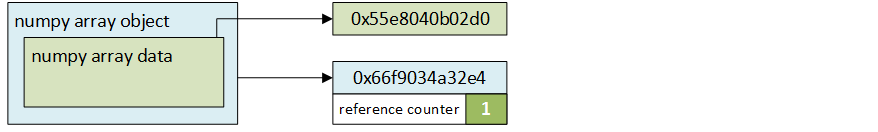(a) The NumPy array is created in Python. Note that the NumPy object and the data it contains are represented by two separate memory addresses.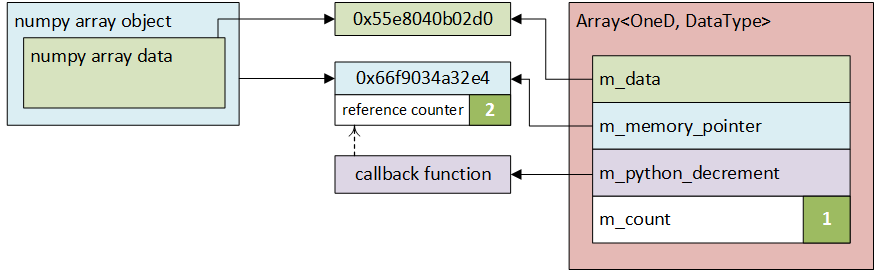(b) The C++ array is created through the converter method: its attributes point to the appropriate memory addresses and the reference counter of the memory address of NumPy array object is incremented.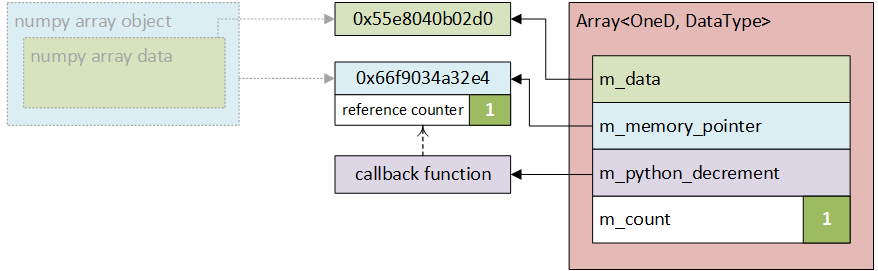(c) If the NumPy object is deleted first, the reference counter is decremented, but the data still exists in memory.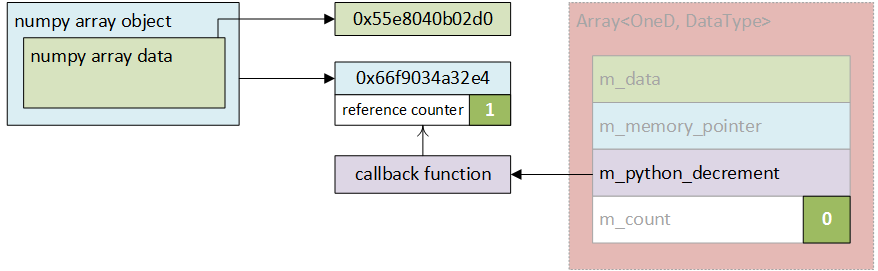(d) If the C++ array is deleted first, the callback function decrements the reference counter of the NumPy object but the data still exists in memory.

Figure 23.4 Diagram showing the process of creation and deletion of arrays. Reference counters shown in green.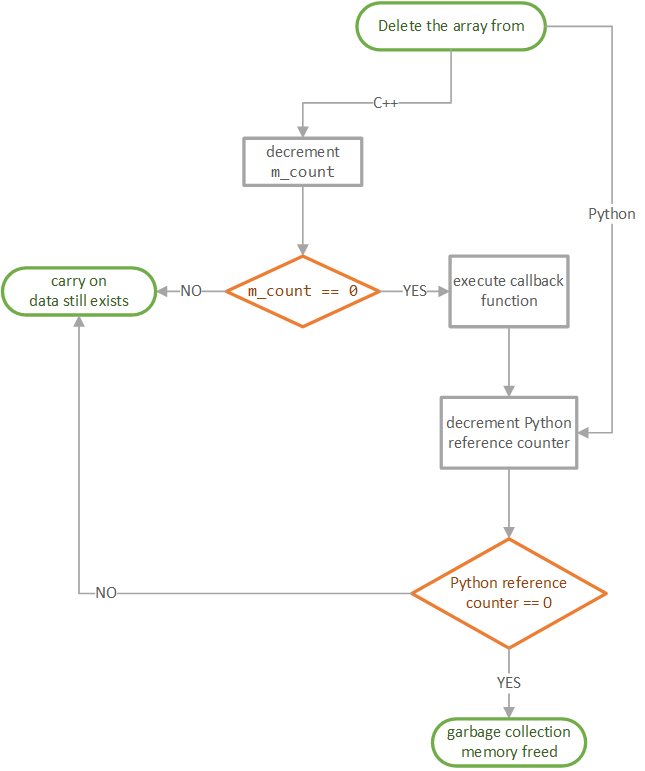Figure 23.5 Flowchart describing the procedure for array deletion from either side of the code.

##### Testing

As the process of converting arrays from Python to C++ required making direct calls to C API and relying on custom-written methods, more detailed testing was deemed necessary. In order to thoroughly check if the conversion works as expected, three tests were conducted to determine whether the array is:

1. referenced (not copied) between the C++ program and the Python wrapper,
2. accessible to C++ program after being deleted in Python code,
3. accessible in Python script after being deleted from C++ objects.

Python files containing test scripts are currently located in library\Demos\Python\tests. They should be converted into unit tests that should be run when Python components are built.